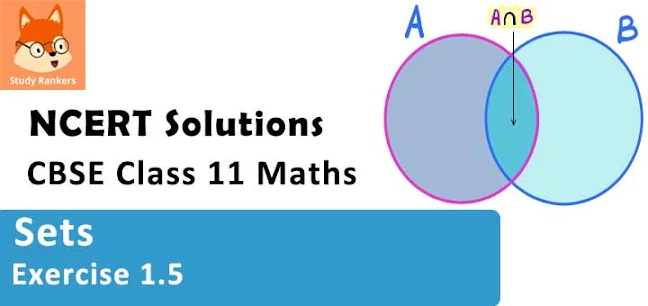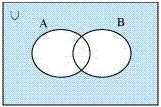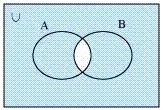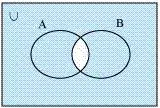# Class 11 Maths NCERT Solutions for Chapter 1 Sets Exercise 1.5### Sets Exercise 1.5 Solutions

1. Let U ={1, 2, 3; 4, 5, 6, 7, 8, 9}, A = {1, 2, 3, 4}, B = {2, 4, 6, 8} and C = {3, 4, 5, 6}. Find
(i) A'
(ii) B'
(iii) (A ∪ C)'
(iv) (A ∪ B)'
(v) (A')'
(vi) (B - C)'

Solution

U ={1, 2, 3, 4, 5, 6, 7, 8, 9}
A = {1, 2, 3, 4}
B = {2, 4, 6, 8}
C = {3, 4, 5, 6}
(i) A' = {5, 6, 7, 8, 9}
(ii) B' = {1, 3, 5, 7, 9}
(iii) A ∪ C = {1, 2, 3, 4, 5, 6}
∴ (A∪ C)' = {7, 8, 9}
(iv) A ∪ B = { 1, 2, 3, 4, 6, 8}
(A∪ B)' = { 5, 7, 9}
(v) (A')' = A = { 1, 2, 3, 4}
(vi) B - C = { 2, 8 }
∴ (B - C)' = { 1, 3, 4, 5, 6, 7, 9}

2. If U = {a, b, c, d, e, f, g, h}, find the complements of the following sets:
(i) A = {a, b, c}
(ii) B = {d, e, f, g}
(iii) C = {a, c, e, g}
(iv) D = {f, g, h, a}

Solution

U = {a, b, c, d, e, f, g, h}
(i) A = {a, b, c}
A’ = {d, e, f, g, h}
(ii) B = {d, e, f, g}
∴ B’ = {a, b, c, h}
(iii) C = {a, c, e, g}
∴C’ = { b, d, f, h}
(iv) D = {f, g, h, a}
∴ D’ = { b, c, d, e}

3. Taking the set of natural numbers as the universal set, write down the complements of the following sets:
(i) {x: x is an even natural number}
(ii) {x: x is an odd natural number}
(iii) {x: x is a positive multiple of 3}
(iv) {x: x is a prime number}
(v) {x: x is a natural number divisible by 3 and 5}
(vi) {x: x is a perfect square}
(vii) {x: x is perfect cube}
(viii) {x: x + 5 = 8}
(ix) {x: 2x + 5 = 9}
(x) {x: x ≥ 7}
(xi) {x: x ∈ N and 2x + 1 > 10}

Solution

U = N: Set of natural numbers
(i) {x: x is an even natural number}´ = {x: x is an odd natural number}
(ii) {x: x is an odd natural number}´ = {x: x is an even natural number}
(iii) {x: x is a positive multiple of 3}´ = {x: x ∈ N and x is not a multiple of 3}
(iv) {x: x is a prime number}´ ={x: x is a positive composite number and x = 1}
(v) {x: x is a natural number divisible by 3 and 5}´ = {x: x is a natural number that is not divisible by 3 or 5}
(vi) {x: x is a perfect square}´ = {x: x ∈ N and x is not a perfect square}
(vii) {x: x is a perfect cube}´ = {x: x ∈ N and x is not a perfect cube}
(viii) {x: x + 5 = 8}´ = {x: x ∈ N and x ≠ 3}
(ix) {x: 2x + 5 = 9}´ = {x: x ∈ N and x ≠ 2}
(x) {x: x ≥ 7}´ = {x: x ∈ N and x < 7}
(xi) {x: x ∈ N and 2x + 1 > 10}´ = {x: x ∈ N and x ≤ 9/2}

4. If U = {1, 2, 3, 4, 5,6,7,8, 9}, A = {2, 4, 6, 8} and B = {2, 3, 5, 7}. Verify that
(i)  (A ∪ B)' = A' ∩ B'
(ii)  (A ∩ B)' = A' ∪ B'

Solution

U = {1, 2, 3, 4, 5, 6, 7, 8, 9}
A = {2, 4, 6, 8}, B = {2, 3, 5, 7}

(i) (A ∪ B)ʹ = {2, 3, 4, 5, 6, 7, 8} = {1, 9}
Aʹ ∩ Bʹ = {1, 3, 5, 7, 9} ∩ {1, 4, 6, 8, 9} = {1, 9}
So, (A ∪ B)ʹ = Aʹ ∩ Bʹ

(ii) (A ∩ B)' = {2}' = {1, 3, 4, 5, 6, 7, 8, 9}
A'∪ B' = {1, 3, 5, 7, 9} ∪ {1,4, 6, 8, 9} = {, 1, 3, 4, 5, 6, 7, 8, 9}
∴ (A ∩ B)' = A' ∪ B'

5. Draw appropriate Venn diagram for each of the following :
(i) (A ∪ B)'
(ii) A' ∩ B'
(iii) (A∩B)'
(iv) A' ∪ B'

Solution

(i) (A ∪ B)'(ii) A' ∩ B'(iii) (A∩B)'(iv) A' ∪ B'6. Let U be the set of all triangles in a plane. If A is the set of all triangles with at least one angle different from 60°, what is A' ?

Solution

A' is the set of all equilateral triangles.

7. Fill in the blanks to make each of the following a true statement:
(i) A ∪ A ' =  .....
(ii) Φ ∩ A =  .....
(iii) A ∩ A' = .....
(Iv) U' ∩ A =  ......

Solution

(i) A ∪ A ' = U
(ii) Φ′ ∩ A = U ∩ A = A
∴ Φ′ ∩ A = A
(iii) A ∩ A′ = Φ
(iv) U′ ∩ A = Φ ∩ A = Φ
∴ U′ ∩ A = Φ